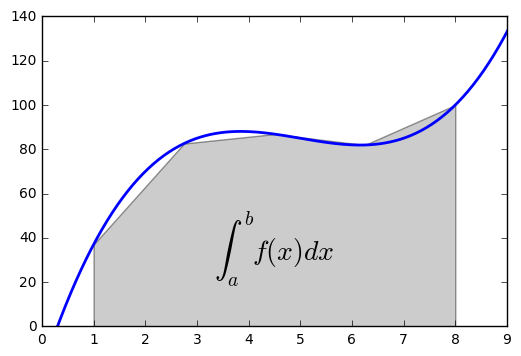In :
# coding: utf-8

# # Basic Numerical Integration: the Trapezoid Rule

# A simple illustration of the trapezoid rule for definite integration:
#
# $$#$$
# <br>
# First, we define a simple function and sample it between 0 and 10 at 200 points

# In:

get_ipython().magic('matplotlib inline')
import numpy as np
import matplotlib.pyplot as plt

# In:

def f(x):
return (x-3)*(x-5)*(x-7)+85

x = np.linspace(0, 10, 200)
y = f(x)

# Choose a region to integrate over and take only a few points in that region

# In:

a, b = 1, 8 # the left and right boundaries
N = 5 # the number of points
xint = np.linspace(a, b, N)
yint = f(xint)

# Plot both the function and the area below it in the trapezoid approximation

# In:

plt.plot(x, y, lw=2)
plt.axis([0, 9, 0, 140])
plt.fill_between(xint, 0, yint, facecolor='gray', alpha=0.4)
plt.text(0.5 * (a + b), 30,r"$\int_a^b f(x)dx$", horizontalalignment='center', fontsize=20);

# Compute the integral both at high accuracy and with the trapezoid approximation

# In:

from __future__ import print_function

The integral is: 565.25 +/- 6.27553564669e-12